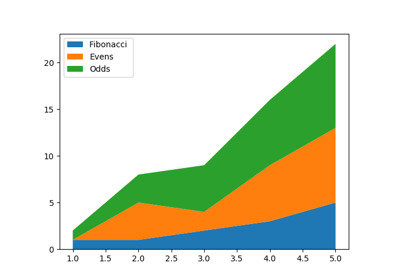# matplotlib.axes.Axes.stackplot¶

Axes.stackplot(axes, x, *args, labels=(), colors=None, baseline='zero', data=None, **kwargs)

Draw a stacked area plot.

Parameters: x1d array of dimension N y2d array (dimension MxN), or sequence of 1d arrays (each dimension 1xN)The data is assumed to be unstacked. Each of the following calls is legal: stackplot(x, y) # where y is MxN stackplot(x, y1, y2, y3, y4) # where y1, y2, y3, y4, are all 1xNm  baseline{'zero', 'sym', 'wiggle', 'weighted_wiggle'}Method used to calculate the baseline: 'zero': Constant zero baseline, i.e. a simple stacked plot. 'sym': Symmetric around zero and is sometimes called 'ThemeRiver'. 'wiggle': Minimizes the sum of the squared slopes. 'weighted_wiggle': Does the same but weights to account for size of each layer. It is also called 'Streamgraph'-layout. More details can be found at http://leebyron.com/streamgraph/. labelsLength N sequence of stringsLabels to assign to each data series. colorsLength N sequence of colorsA list or tuple of colors. These will be cycled through and used to colour the stacked areas. **kwargsAll other keyword arguments are passed to Axes.fill_between(). listlist of PolyCollectionA list of PolyCollection instances, one for each element in the stacked area plot.

Notes

Note

In addition to the above described arguments, this function can take a data keyword argument. If such a data argument is given, the following arguments are replaced by data[<arg>]:

• All positional and all keyword arguments.

Objects passed as data must support item access (data[<arg>]) and membership test (<arg> in data).

## Examples using matplotlib.axes.Axes.stackplot¶